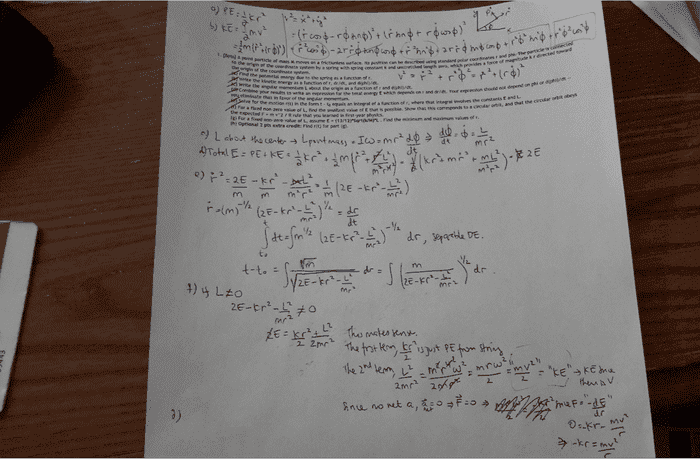# Classical Mechanics - Energy+Circular Motion problem

## Homework Statement

A point particle of mass m moves on a frictionless surface. Its position can be described with polar coordinates r and phi. The particle is connected to the origin of the coordinate system by a spring with spring constant k and unstretched length zero, which provides a force of magnitude k r directed toward the origin of the coordinate system.
(a) Find the potential energy due to the spring as a function of r.
(b) Write the kinetic energy as a function of r, dr/dt, and d(phi)/dt.
(c) Write the angular momentum L about the origin as a function of r and d(phi)/dt.
(d) Combine your results to write an expression for the total energy E which depends on r and dr/dt. Your expression should not depend on phi or d(phi)/dt -- you eliminate that in favor of the angular momentum.
(e) Solve for the motion r(t) in the form t - t0 equals an integral of a function of r, where that integral involves the constants E and L.
(f) For a fixed non-zero value of L, find the smallest value of E that is possible. Show that this corresponds to a circular orbit, and that the circular orbit obeys the expected F = m v^2 / R rule that you learned in first-year physics.
(g) For a fixed non-zero value of L, assume E = (13/12)*Sqrt(k/M)*L . Find the minimum and maximum values of r.

## Homework Equations

See "attempt..." below

## The Attempt at a Solution

part a. PE in this case is kr^2/2 (due to spring only)
part b. KE = mv^2/2, where v is r_dot^2+(r*phi_dot)^2, see attachment for how I derived this--I apologize for not being familiar with the formatting here!

L=Iw=mr^2w, w=d(phi)/dtheta

what I did: part c->solve for d(phi)/dtheta
part d->use d(phi)/dtheta from part c to replace the same thing in KE equation. Add KE and PE=Total E
part e-> solve for r_dot. Then make it into separable DE, solve the left side (integral dt=t-t_0) and it equals to a crazy right hand side below
part f->set 2E-k^r2-L^2/(m*r^2) to 0 because it can't be 0, or otherwise there is a problem. checked it and it llokd OK
(-----part g->(not sure part) set r_dot=0, solve for r, except I couldn't. I know that I should be getting 2 r values, but wolfram spits out imaginary answer. And I need to find r_dot_dot, plug in the r values. If positive=minima, negative=maxima (my plan, anyway)------)

I have work for part a-f, currently stuck in part g. And now I question my whole work.(here is the link, just in case the picture doesn't show: http://i58.tinypic.com/30hlp1s.jpg)

Thank you very much in advance. Any enlightenment or confirmation if I am in the right direction or suggestion or advice would be very much appreciated.